[BACK]Computer Modeling in Engineering & SciencesDOI: 10.32604/cmes.2022.020598

ARTICLE

Group Decision-Making Method with Incomplete Intuitionistic Fuzzy Preference Relations Based on a Generalized Multiplicative Consistent Concept

1School of Computer Science, Qinghai Normal University, Xining, 810008, China
2School of Science, Shanghai Institute of Technology, Shanghai, 201418, China
3School of Statistics, Jiangxi University of Finance and Economics, Nanchang, 330013, China
4School of Information Technology, Jiangxi University of Finance and Economics, Nanchang, 330013, China
Corresponding Author: Jiuying Dong. Email: jiuyingdong@126.com
Received: 02 December 2021; Accepted: 26 January 2022

Abstract: Based on the analyses of existing preference group decision-making (PGDM) methods with intuitionistic fuzzy preference relations (IFPRs), we present a new PGDM framework with incomplete IFPRs. A generalized multiplicative consistent for IFPRs is defined, and a mathematical programming model is constructed to supplement the missing values in incomplete IFPRs. Moreover, in this study, another mathematical programming model is constructed to improve the consistency level of unacceptably multiplicative consistent IFPRs. For group decision-making (GDM) with incomplete IFPRs, three reliable sources influencing the weights of experts are identified. Subsequently, a method for determining the weights of experts is developed by simultaneously considering three reliable sources. Furthermore, a targeted consensus process (CPR) is developed in this study with reference to the actual situation of the consensus level of each IFPR. Meanwhile, in response to the proposed multiplicative consistency definition, a novel method for determining the optimal priority weights of alternatives is redefined. Lastly, based on the above theory, a novel GDM method with incomplete IFPRs is developed, and the comparative and sensitivity analysis results demonstrate the utility and superiority of this work.

Keywords: Intuitionistic fuzzy preference relations; multiplicative consistency; consensus; optimization model; group decision-making

1  Introduction

Multi-attribute decision-making (MADM) aims to identify the best alternative from a set of finite feasible alternatives by considering multiple attributes that are used extensively in numerous fields of modern life . However, because of the many evaluation factors of complex decision-making problems, it is difficult for experts to provide a corresponding evaluation value for each factor, leading to the emergence of preference DMs (PDMs) [4,5]. The PDM is used to identify the best alternative from a set of finite feasible alternatives using preference relations (PRs) between alternatives offered by experts, which is simpler and more convenient than MADM. PR is a form of pairwise comparison between alternatives, and was initially reported by Saaty  in the analytic hierarchy process. Since PRs are highly consistent with people’s evaluation habits and experts can provide them easily, consequently, PDM has received widespread attention in recent years. Currently, there are two primary types of PRs, i.e., additive preference relations  and multiplicative preference relations . With extensive research on PDM, many derivatives of the two PRs have emerged through the intervention of various fuzzy sets, such as interval fuzzy preference relations (IVFPRs) [9,10], intuitionistic fuzzy preference relations (IFPRs) , intuitionistic multiplicative preference relations (IMPRs) , complex intuitionistic fuzzy preference relations (CIFPRs) , hesitant fuzzy preference relations (HFPRs) , probabilistic hesitant fuzzy preference relations (PHFPRs) , etc. . Among them, since IFPRs can express the pairwise comparison between alternatives from the following three dimensions: affirmative, negative, and hesitant, IFPRs are more popular in group decision-making (GDM) when expressing the pairwise comparison between alternatives with uncertain information. Therefore, GDM with IFPRs is an interesting topic. Generally, it is necessary to perform three processes for GDM with IFPRs. First, the consistency of IFPRs is an essential issue in GDM, the quality of which directly affects the reasonability and acceptability of the final DM result. Researches related to the consistency of IFPRs in GDM includes how to define, check, and repair the consistency. Second, the consensus among individual IFPRs is another crucial issue in GDM with IFPRs, the quality of which directly impacts the recognition and acceptance of the final DM result by all members in the group. Researches related to consensus in GDM with IFPRs includes checking and improving the consensus. In addition to the above two issues, the ranking process of alternatives is the third issue that must be solved in GDM with IFPRs. Research on the ranking process of alternatives primarily refers to the selection of a reasonable method for determining the priority weight vector of the alternatives. Although many achievements have been made in the research on GDM with IFPRs, these methods are imperfect for numerous reasons. Therefore, this study continued to study GDM with IFPRs.

The remainder of the paper is organized as follows. Section 2 briefly recalls the literature review of GDM with IFPRs. This section also resumes the research motivations, novelties and research methodology of this study. In Section 3, the concepts regarding IFPRs are reviewed. In Section 4 a generalized multiplicative consistency definition of IFPRs is presented. Section 5 constructs respectively two mathematically programming models to supplement the default values in incomplete IFPRs and improve the consistency level of the unacceptably consistent IFPRs. After that develops a new consistency improving method with incomplete IFPRs. Section 6 introduces a new method of generating the experts’ weights, and then designs a targeted CPR and develops a method determining priority weight vector of alternatives. Further, based on the above theoretical basics, a new GDM method with incomplete IFPRs is developed. In Section 7, an application example is supplied to verify the practicability of the method presented in this study, comparative and sensitivity analysis result shows the advantages of this method. Concluding remarks are given in Section 8.

2  Literature Review

As an extension of the conventional fuzzy set portrayed by Atanassov , the intuitionistic fuzzy set (IFS) theory uses three-dimensional (3D) degrees to characterize the experts’ positive, negative, and indeterminate cognitions. Owing to its robust advantages in describing the uncertainty and vagueness of human thinking, the IFS has received special attention in the field of DM. Therefore, many DM methods based on the IFS have also been developed. For instance, Liao et al. manifested the intuitionistic fuzzy Preference Ranking Organization Method for Enrichment Evaluations (PROMETHEE) , Liao et al.  extended Analytic Network Process (ANP) into intuitionistic fuzzy environments and developed the intuitionistic ANP (IFANP) method, and Wan et al.  introduced the Best-worst method with IFPRs, and Efe  proposed IF modelling-based risk assessment approaches using IF numbers-based quality function deployment (QFD) and VIseKriterijumska Optimizacija I Kompromisno Resenje (VIKOR) technique. Stanujkić et al.  proposed a new extension of the WASPAS method for DM problems with intuitionistic fuzzy numbers. Moreover, Kushwaha et al.  presented an integrated framework based on Intuitionistic Fuzzy-Failure Mode Effect Analysis (IF-FMEA) and IF-Technique for Order Preference by Similarity to Ideal Solution (IF-TOPSIS) techniques to rank the listed failure causes, etc. Among the methods based on IFS for handling DM problems, the DM method based on IFPRs is one of the fundamental and efficient methods for modeling DM issues. In recent years, many achievements have been made regarding DM with IFPRs. Xu et al.  developed a consensus analysis method for GDM with IFPRs based on a defined similarity measure between IFSs. Xu et al.  first presented the multiplicative consistency definition and used it to estimate probable missing elements in an incomplete IFPR, after which they developed a GDM method with incomplete IFPRs. Based on the consistency definition , Xu et al.  developed an approach to enhance the consistency level of unacceptably consistent IFPRs and extended the classic analytic hierarchy process (AHP) into intuitionistic fuzzy AHP (IFAHP). Furthermore, Liao et al.  found that the multiplicative consistency condition proposed in  conflicts with Saaty’s multiplicative transitivity property because of stringent constraints and proposed a general multiplicative consistency definition of IFPRs and developed a novel approach to derive the priority weights from an IFPR. Based on the multiplicative consistency definition presented in , Liao et al.  devised an iterative algorithm to enhance the consistency level of IFPRs, and then presented an interesting consensus reaching process, and Xu et al.  constructed an optimization model to repair the consistency of the unacceptably consistent IFPRs and developed a GDM method with IFPRs by simultaneously considering the acceptable consensus and consistency. Jin et al.  developed a novel approach to derive the priority weights from an IFPR and proposed a GDM method with IFPRs. In addition, according to the isomorphism between IVFPRs and IFPRs, Gong et al.  introduced the definition of multiplicative consistent IFPR, based on which the intuitionistic fuzzy priority weight vector (IFPWV) was produced through some goal programming models. Moreover, Wu et al.  initiated multiplicative consistency of IFPRs by a proposed multiplicative consistency definition for IVFPRs, and designed a consistency and consensus improving method based on feedback mechanism. Based on the multiplicative consistency of IFPRs presented in , Wu et al.  constructed a multiplicative consistency-based multi-objective programming (MOP) model to derive the priority vector from an IFPR. More recently, Meng et al.  provided an innovative multiplicative consistency definition of IFPRs based on the quasi-interval-based transitivity equation of IVFPRs and constructed a consensus model to enhance consensus among experts. Based on Krejčí  multiplicative consistency definition of IVFPRs, Meng et al.  redefined the multiplicative consistency of IFPRs, and Wang  presented a novel multiplicative consistency definition of IFPRs and developed a representable uninorm based IFAHP.

2.1 Research Motivations

The literature reviews reveal that the above mentioned achievements are effective in dealing with real DM problems; however, there are some gaps in the following aspects, which can be summarized as follows:

(1) The first gap obtained from existing studies is in terms of consistency management in GDM with IFPRs. In , the multiplicative consistency of individual IFPRs was not considered, which could lead to an unreasonable DM result. In , Wang pointed out that these multiplicative consistency definitions for IFPRs presented in [25,27,32] are not intuitionistic fuzzy extensions of Tanino’s multiplicative consistency. Therefore, the DM outcomes obtained by GDM methods [28,29,32], which were respectively developed based on the consistency definitions in [25,27,32] may lack rationality and persuasiveness. Moreover, because the GDM method with IFPRs  is developed based on complete multiplicative consistency, and the DM result is produced at the cost of a large amount of original information loss (it can be seen from the comparative analysis in Section 6). For GDM methods [30,31], the consistency of the IFPRs has not been considered. Given the shortcomings of consistency management in GDM with IFPRs [25,2729,32,34], it is essential to present a more reasonable consistency management framework for GDM with IFPRs, which is the first motivation of this study.

(2) The second gap obtained from existing studies is in terms of the method of determining the weights of experts. In these methods [24,25,28,30,31], the experts’ weights are assigned in advance, which makes it difficult to avoid subjective randomness. In , the experts’ weights were derived by simultaneously considering the consistency index and confidence degree. In , experts’ weights were derived by considering the consistency index. In , experts’ weights were derived by considering the degree of confidence. Although these methods determine experts’ weights in [29,32,34] effectively avoid the subjective randomness of the experts’ weights, they only consider one or two aspects affecting experts’ weights. In fact, some factors, such as the hesitancy degree of the IFPRs and the ability to retain the original information, have a greater impact on the experts’ weights. In view of this fact, it is essential to propose a method determining the experts’ weights that comprehensively considers these aspects, which is the second motivation of this research.

(3) The third gap obtained from existing studies is in terms of the consensus-reaching process (CRP) in GDM with IFPRs. In the methods [25,30,31], group consensus among experts was not considered. In [24,28,32,34], the information loss of the original individual IFPRs was ignored in CRP. In , although the information loss of the original individual IFPRs has gained attention, the adjustments for individual IFPRs were accomplished by modifying all individual IFPRs via an optimization model, which illustrates the adjustment without the target. Thus, the final adjusted individual IFPRs may not be optimal in terms of preserving the original information (it is evident from the comparative analysis in Section 6). In view of the shortcomings of the CRP proposed in [24,2832,34], it is essential to develop a more reasonable consensus-improving method, which is the third motivation of this study.

(4) The fourth gap obtained from existing studies exist in terms of the method employed for obtaining the priority weights of alternatives. In the methods [25,28,29,32,34], the priority weights of alternatives have been primarily determined by some aggregation operator; however, for some situations, it may cause a number of information losses if this method is adopted. However, although the priority weights of alternatives are derived by the optimization model in [27,30,31], which effectively prevents the loss of information, the DM result may lack persuasiveness because the priority weights of alternatives are generated based on the group IFPR without checking the consistency. In view of the shortcomings of methods for obtaining the priority weights of alternatives in [25,2732,34], it is necessary to develop a more reasonable method to obtain the priority weights of alternatives, which is the fourth motivation of this study.

To bridge the above four research motivations, this study aims to develop a novel GDM method using IFPRs, the key novelties of which are listed below:

(1) A more generalized multiplicative consistency definition for IFPRs is presented, which compensates for the defects of the existing multiplicative consistency definitions for IFPRs. Furthermore, a more reasonable consistency management framework for GDM with IFPRs is presented, in which the acceptable multiplicative consistency of individual IFPRs is considered.

(2) Three reliable sources influencing experts’ weights were first identified by analyzing the factors affecting experts’ weights, and a method of determining experts’ weights was developed by simultaneously considering three reliable sources.

(3) A targeted consensus-improving method is developed by which the individual IFPRs obtained retain the original information to the greatest extent while achieving an acceptable consensus and consistency level.

(4) The priority weights of the alternatives were derived from the acceptably multiplicative consistent IVFPR via the optimization model, which prevents information loss and enhances the persuasiveness of the obtained DM result.

2.3 Research Methodology

To achieve the goal mentioned above, the research methodology for the present study is shown in Fig. 1.Figure 1: Research methodology

Stage 1: Consistency improving phase: Based on the proposed multiplicative consistency definition for IFPRs, two mathematical programming models are respectively constructed to supplement the default values in incomplete IFPRs and improve the consistency level of unacceptably consistent IFPRs.

Stage 2: Consensus improving phase: This stage introduces a new method of generating experts’ weights, and then designs a targeted consensus improving method to enhance the consensus level of individual IFPRs.

Stage 3: Exploitation phase: Based on the proposed multiplicative consistency definition for IFPRs, a method determining the priority weights of alternatives is developed.

3  Preliminaries

This section mainly reviews some relevant concepts of IFSs and IFPRs. Let N={1,2,,n} and Q={1,2,,q} be the corresponding sets of indices.

Definition 3.1.  Assume that Z={z1,z2,,zn} is a fixed set, an IFS in Z is defined as T={<z,μT(z),υT(z)>|zZ}, where μT(z) and υT(z) denote the membership and non-membership degrees of zZ to set T, respectively, such that μT(z),υT(z)[0,1] and μT(z)+υT(z)1 for zZ. πT(z)=1μT(z)υT(z) is called the hesitancy degree of zZ to set T.

In addition, Xu et al.  introduced the intuitionistic fuzzy number (IFN), which is generally denoted as a=(μa,υa), where 0μa,υa1 and μa+υa1.

Definition 3.2.  Assume that a1=(u1,ν1) and a2=(u2,ν2) are two IFNs, the distance between a1 and a2 is defined as Eq. (1):

d(a1,a2)=12(|u1u2|+|ν1ν2|)(1)

Definition 3.3.  Let X be a finite set of compared alternatives. An IFPR A on X is represented by a matrix A=(aij)n×n=(μij,υij)n×n, where μij and υij denote the membership and non-membership degrees to which xi is preferred to xj, respectively. Moreover, μij and υij satisfy: μij,υij[0,1], μij=υji, υij=μji, μij+υij1, μii=υii=1, for all i,jN.

4  A New Multiplicative Consistency Framework for IFPRs

In this section, based on the multiplicative consistency definition for IFPRs proposed by Meng et al. , a new consistency index for IFPRs is first defined, after which the corresponding acceptably multiplicative consistency definition for IFPRs is presented.

Definition 4.1.  Let A=(aij)n×n=(μij,υij)n×n be an IFPR. If for any preferred degree εij[μij,1υij], there always exist two preferred degrees εik[μik,1υik] and εkj[μkj,1υkj], such that the three preferred degrees εij, εik and εkj satisfy Tanino’s multiplicative transitivity , i.e., Eq. (2):

εijεji=εikεkiεkjεjk(i,k,jN;ikj)(2)

Then A is regarded as multiplicative consistent.

Property 4.1.  Let A=(aij)n×n=(μij,υij)n×n be an IFPR. If A satisfies the following inequalities:

μij1υjiμik1υkiμkj1υjk,1υijμji1υikμki1υkjμjk(i,k,jN;ki,j;i<j)(3)

Then A is regarded as a multiplicative consistent IFPR.

Since μji=υij and υji=μij, Eq. (3) can be reduced as:

1υijυij1υikυik1υkjυkj(i,k,jN;ikj)(4)

By taking the logarithm on both sides of Eq. (4), we can obtain

log(1υij)logυijlog(1υik)logυik+log(1υkj)logυkj(i,k,jN;ikj)(5)

It should be noted that Eq. (5) holds only when the IFPR A is completely consistent. However, in the real DM problems, it is not easy for an expert to offer a completely consistent IFPR, that is to say, it is difficult to find an IFPR meeting Eq. (5). Given the above facts, the smaller deviations are usually permitted. Thus, we expect to seek an IFPR to satisfy Eq. (5) in fuzzy sense, i.e., Eq. (6):

log(1υij)logυij(log(1υik)logυik+log(1υkj)logυkj)~0(i,k,jN;ikj)(6)

where the symbol “~” is the fuzzy version for symbol “” in the real number and has the linguistic interpretation “essentially equal to”. Let

Raikj=log(1υij)logυij(log(1υik)logυik+log(1υkj)logυkj)(i,k,jN;ikj)(7)

then Eq. (6) can be rewritten as Raikj ~0 (i,k,jN;ikj). Furthermore, we construct the linear membership function to represent the intensity of satisfaction for fuzzy constraints given in Eq. (7) as the following Eq. (8):

M(Raikj)={0Raikj>tikj1Raikjtikj0Raikjtikj1Raikj<0(i,k,jN;ikj)(8)

where tikj(i,k,jN;ikj) are tolerance parameters.

In what follows, based on the above linear membership functions for fuzzy constraint given in Eq. (7), a new multiplicative consistency index to quantify the multiplicative consistency level of IFPRs is presented.

Definition 4.2. Let A=(aij)n×n=(μij,υij)n×n be an IFPR. The multiplicative consistency index MCI(A) of the IFPR A is defined as the following Eq. (9):

MCI(A)=i=1nj=1,jink=1,ki,jnμ(M(Raikj))n×(n1)×(n2)(9)

where μ(M(Raikj))=min{max{M(Raikj),0},1}(i,k,jN;ikj) represent the linear memberships for fuzzy constraints given in Eq. (6).

Remark 4.1. It is apparent that 0MCI(A)1. The bigger the value of MCI(A), the better consistency of A. Specifically, if MCI(A)=1, then A is called a completely multiplicative consistent IFPR.

Definition 4.3. Let MCI0[0,1] be a consistent threshold. If MCI(A)MCI0, then A is regarded as an acceptably multiplicative consistent IFPR. Otherwise, it is called an unacceptably one.

However, due to the complexity and uncertainty of DM problems involved in real life, it is not an easy thing for experts to provide acceptably consistent IFPRs. For these unacceptably consistent IFPRs, generally, the judgments of IFPRs needs to be adjusted by establishing related optimization model to make the IFPRs reach an acceptable consistency level.

5  A New Method for Improving the Consistency of the Incomplete IFPRs

It is common to encounter information default in DM because of experts’ limited expertise and time pressure or the complex DM environment. Therefore, it is very necessary to present an effective method to deal with the DM problems with incomplete information under intuitionistic fuzzy context. To do this, we first need to supplement these missing values. In this section, based on the above multiplicative consistency measure concept, the missing values in an incomplete IFPR are estimated by constructing related optimization model.

5.1 Optimization Model for Estimating Missing Values in Incomplete IFPRs

Before constructing the optimization model to estimate missing values in incomplete IFPRs, the relevant concepts for incomplete IFPRs are first introduced in the following.

Definition 5.1. Let A=(aij)n×n=(μij,υij)n×n be an IFPR. If some membership degrees, non-membership degrees or both membership and non-membership degrees of its pairwise judgments are missing, then A is regarded as an incomplete IFPR.

In this paper, A~=(a~ij)n×n=(μ~ij,υ~ij)n×n is employed to denote the incomplete IFPR to distinguish the complete IFPR A. As to A~, let Nij={(i,j)|μ~ij is unknown i,jN} and Nμ={(i,k,j)|μ~ijμ~ikμ~kj is unknown, i,k,jN}.

Definition 5.2. Let A~=(a~ij)n×n be an incomplete IFPR. If there exist some values in [0,1] for all unknown values in Nμ satisfying Eq. (4), then the incomplete IFPR A~ is multiplicative consistent.

In fact, for an incomplete IFPR A~, we often cannot guarantee that Eq. (4) holds. That is to say, the membership value for fuzzy constraints given in Eq. (6) may be not equal to 1. Thus, we often seek some values in [0,1] for all unknown values in Nμ to make the membership value for fuzzy constraints given in Eq. (6) reach the maximum. Given the above facts, we construct the following mathematical programming model:

max(i,k,j)Nμμ(M(Ra~ikj))s.t.{min{max{M(Ra~ikj),0},1}=μ(M(Ra~ikj))μ~ij0,μ~ij1υ~ij(i,j)Nijμ~ij0,μ~ji0,μ~ij+μ~ji1(i,j)Nij(j,i)Nji(10)

where M(Ra~ikj) are derived by Eq. (8). The first constrain comes from Definition 4.2, and the rest constrains ensure that the supplemented values for A~ meet the requirement of IFNs. By solving the Eq. (10), we obtain the optimal solutions, denoted by μ~ij(i,j)Nij. Furthermore, based on these optimal solutions, the complete IFPR A associated with A~ is derived.

5.2 Optimization Model for Improving Consistency Level of Complete IFPRs

The consistency level of PR has a crucial impact on the final DM result. It happens too often that an unreasonable DM result may be generated from a PR with poor consistency. In fact, a complete IFPR A given by expert (or derived by solving Eq. (10)) is generally unacceptably consistent, namely, MCI(A)<MCI0. To acquire an acceptably consistent IFPR A¯=(a¯ij)n×n=(μ¯ij,υ¯ij)n×n associated with A, a mathematical programming model is constructed as follows:

=min1n(n1)i=1n1j=i+1n(|μijμ¯ij|+|υijυ¯ij|)s.t.{min{max{M(Ra¯ikj),0},1}=μ(M(Ra¯ikj))MCI(A¯)=i=1nj=1,jink=1,ki,jnμ(M(Ra¯ikj))n×(n1)×(n2)MCI00μ¯ij,υ¯ij1,μ¯ij+υ¯ij1,μ¯ij=υ¯ji,υ¯ij=μ¯ji(11)

where M(Ra¯ikj) are derived by Eq. (8). The first two constrains come from Definition 4.2, which ensure that the obtained IFPR is acceptably consistent. The third constrain ensures that the obtained matrix is an IFPR.

To solve Eq. (11) conveniently, let ϕij+=(|μijμ¯ij|+(μijμ¯ij))2, ϕij=(|μijμ¯ij|(μijμ¯ij))2, φij+=(|υijυ¯ij|+(υijυ¯ij))2, φij=(|υijυ¯ij|(υijυ¯ij))2, then Eq. (11) can be converted into a liner program model as:

=min1n(n1)i=1n1j=i+1n(ϕij++ϕij+φij++φij)s.t.{μijμ¯ij=ϕij+ϕij,υijυ¯ij=φij+φijϕij+0,ϕij0,φij+0,φij0i,jN;i<jother constraints are the same as those of model (11)(12)

By solving Eq. (12), we can obtain the optimal solutions μ¯ij and υ¯ij. Furthermore, according to Definition 3.3, the modified acceptably multiplicative consistent IFPR A¯ associated with A is obtained.

5.3 Algorithm Description for Obtaining the Acceptably Multiplicative Consistent IFPRs

Based on the above theory, a new method for obtaining the acceptably multiplicative consistent IFPRs is brought forward as follows: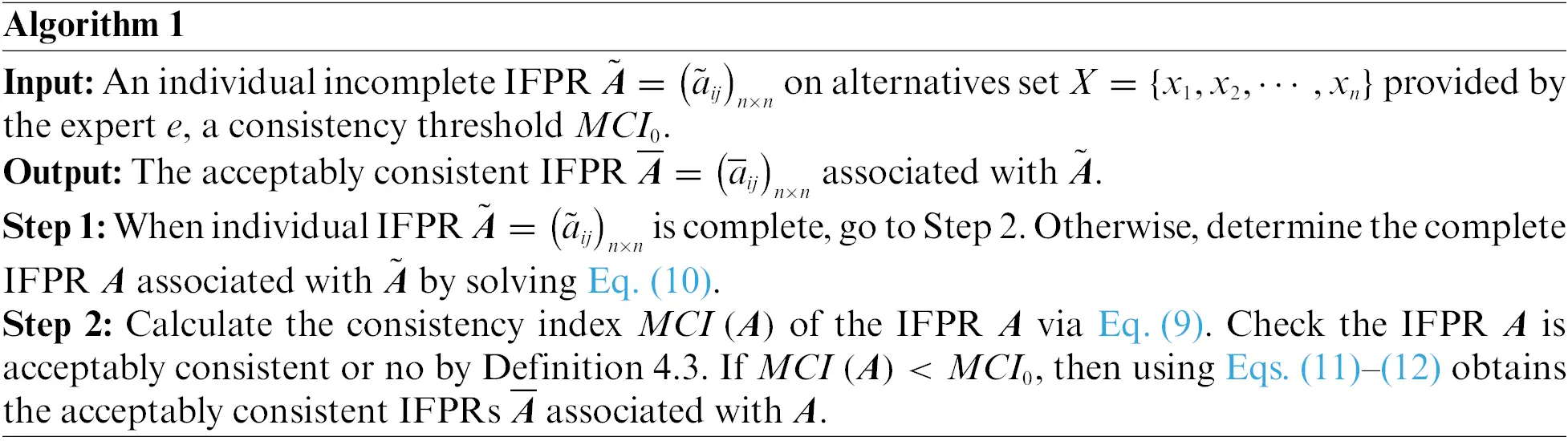6  GDM with Incomplete IFPRs

It is well known that in real life, when facing a complex DM problem, experts or scholars from related fields are usually invited to make decisions collectively, namely, GDM. This section focuses on introducing a new GDM method with incomplete IFPRs. To this end, two parts need to be completed: derivation of experts’ weights and consensus improvement. Without loss of generality, assume that X={x1,x2,,xn} is a set of alternatives that are required for comparison by q experts E={e1,e2,,eq}; A~h corresponds to the incomplete individual IFPRs given by the expert eh(hQ); Ah denotes the complete individual IFPRs associated with A~h obtained by solving Eq. (10); A¯h indicates the acceptably multiplicative consistent IFPR associated with Ah obtained by solving Eqs. (11) and (12).

6.1 Derivation of Experts’ Weights

After obtaining the individual acceptably multiplicative consistent IFPR A¯h=(a¯ij)n×n=(μ¯ij,υ¯ij)n×n(hQ) via Algorithm 1, the next step involves generating the group acceptably multiplicative consistent IFPR A¯C by fusing all individual acceptably multiplicative consistent ones. To achieve this, we must determine the weights of the experts in advance. In the following section, three reliable sources influencing the experts’ weights are identified, after which the experts’ weights are determined by simultaneously considering the three reliable sources.

Generally, the degree of confidence reflects the degree of support from other experts. An expert with a higher confidence degree should be given a larger weight, whereas an expert with a lower confidence degree should be allocated less weight. Moreover, the confidence index CFh of expert eh was calculated using Eq. (13):

CFh=1q1l=1,lhq(1D(A¯h,A¯l))(hQ)(13)

The similarity between the original complete IFPR obtained by solving Eq. (10) (or given by experts) and the acceptably multiplicative consistent IFPR obtained by solving Eq. (11) reflects the degree to which the adjusted IFPR retains original information. More specifically, the larger the deviation, the lesser weight given to the expert, whereas the smaller the deviation degree, the more weight given to the expert. In general, the similarity index between A¯h and Ah was calculated using Eq. (14):

SIh=1D(A¯h,Ah)(hQ)(14)

Furthermore, the hesitancy degree of the IFPR provided by an expert was considered an expert feature. The smaller the hesitancy of the IFPR, the more the weight given to the expert, whereas the bigger the hesitancy of the IFPR, the lesser the weight given to the expert. In general, the hesitancy index of IFPR A¯h is calculated using Eq. (15):

HI(A¯h)=j=in1j=i+1n(1(μ¯ij+υ¯ij))n(n1)(hQ)(15)

Based on the above three reliable sources influencing experts’ weights, a comprehensive index of expert eh is generated as Eq. (16):

CXh=(1HI(A¯h))+SIh+CFh3(hQ)(16)

Let λ=(λ1,λ2,,λq)T be the experts’ weight vector derived from the comprehensive index. Then, λh(hQ) can be calculated as Eq. (17):

λh=CXhh=1qCXh(hQ)(17)

Consensus measures applied to quantify the recognition degree of individual opinions among a group of experts are divided into two types : (1) based on the distance to the group PR  and (2) based on the distance between experts’ PRs . Without loss of generality, the first type is employed in this study to measure the consensus level among individual IFPRs. Prior to presenting the consensus measure concept, we first introduce the calculation of the group IFPRs.

Theorem 6.1.  Let Ah=(aijh)n×n=(μijh,υijh)n×n(hQ) be any q complete IFPRs given by experts eh(hQ) or obtained by solving Eq. (10). The group IFPR AC=(aijC)n×n aggregated by Ah(hQ) is determined as Eq. (18):

aijC=μijC,υijC=h=1q(μijh)λhh=1q(μijh)λh+h=1q(1μijh)λh,h=1q(υijh)λhh=1q(υijh)λh+h=1q(1υijh)λh(i,jN)(18)

where λh[0,1](hQ) is the weight of expert eh(hQ), obtained by Eqs. (13)(17).

Theorem 6.2. Let Ah=(aijh)n×n=(μijh,υijh)n×n(hQ) be q complete IFPRs. If all q IFPRs are acceptably multiplicative consistent, then the group IFPR RC=(rijC)n×n aggregated by Eq. (18) is also acceptably multiplicative consistent.

Proof: As per Eqs. (9) and (18), we have

RaikjC=log(1υijC)logυijC(log(1υikC)logυikC+log(1υkjC)logυkjC)=log(h=1q(1υijh)λh)log(h=1q(υijh)λh)(log(h=1q(1υikh)λh)log(h=1q(υikh)λh)+log(h=1q(1υkjh)λh)log(h=1q(υkjh)λh))=h=1q(λh(log(1υijh)logυijh(log(1υikh)logυikh+log(1υkjh)logυkjh)))=h=1qλhRaikjh

MCI(AC)=i=1nj=1,jink=1,ki,jnμ(M(RaikjC))n×(n1)×(n2)=i=1nj=1,jink=1,ki,jnμ(M(h=1qλhRaikjh))n×(n1)×(n2)=h=1qλhi=1nj=1,jink=1,ki,jnμ(M(Raikjh))n×(n1)×(n2)=h=1qλhMCI(Ah)

Since Ah(hQ) are acceptably multiplicative consistent, one has

MCI(Ah)=i=1nj=1,jink=1,ki,jnμ(M(Raikjh))n×(n1)×(n2)MCI0.

Therefore, MCI(AC)=h=1qλhMCI(Ah)h=1qλhMCI0=MCI0. According to Definition 4.3, AC is acceptably multiplicative consistent.

Remark 6.1. Theorem 6.2 shows that the prerequisite for a group IFPR to be acceptably multiplicative consistent is that all of individual IFPRs used to aggregate into it by Eq. (18) must be acceptably multiplicative consistent. Furthermore, according to Theorem 6. 2, we can also obtain that if all of individual IFPRs are completely multiplicative consistent, then the group IFPR aggregated by these individual IFPRs is completely multiplicative consistent.

6.2 Consensus Improving Process for GDM with IFPRs

In what follows, based on the group IFPR, we first give the concept of consensus measure of the individual IFPRs. Then, a mathematical programming model is constructed to improve the consensus level of individual IFPRs which do not meet the requirement of consensus.

Definition 6.3. Let A¯h=(a¯ijh)n×n=(μ¯ijh,υ¯ijh)n×n(hQ) be q acceptably multiplicative consistent IFPRs, and let A¯C=(a¯ijC)n×n=(μ¯ijC,υ¯ijC)n×n be the group IFPR aggregated by A¯h (hQ) using Eq. (18). Then the consensus index GCM of A¯h is definition as Eq. (19):

GCM(A¯h)=11n(n1)i=1n1j=i+1n(|μ¯ijhμ¯ijC|+|υ¯ijhυ¯ijC|)(hQ)(19)

Clearly, it is 0GCM(A¯h)1, the larger GCM(A¯h), the higher the consensus level of A¯h. Let CM0[0,1] be the consensus threshold. If GCM(A¯t)CM0 for some t=1,2,,ssq, then the consensus level of A¯t(t=1,2,,ssq) need to be adjusted. Without loss of generality, the adjusted IFPR At=(aijt)n×n=(μijt,υijt)n×n corresponding to A¯t (t=1,2,,ssq) is determined as:

{μijt=(μ¯ijt)θij(μ¯ijC)(1θij)(μ¯ijt)θij(μ¯ijC)(1θij)+(1μ¯ijt)θij(1μ¯ijC)(1θij)υijt=(υ¯ijt)θij(υ¯ijC)(1θij)(υ¯ijt)θij(υ¯ijC)(1θij)+(1υ¯ijt)θij(1υ¯ijC)(1θij)θij=θji,θij[0,1](i,jNi<j)(20)

Property 6.1. Let At=(aijt)n×n=(μijt,υijt)n×n be a matrix obtained by Eq. (20). Then the IFPR At is acceptably multiplicative consistent.

Proof: First, we proof At is an IFPR. Since μ¯jit,υ¯ijt,μ¯ijC,υ¯ijC[0,1] (i,jN;t=1,2,,ssq), one has (μ¯ijt)θij(μ¯ijC)(1θij), (υ¯ijt)θij(υ¯ijC)(1θij), (1μ¯ijt)θij(1μ¯ijC)(1θij), (1υ¯ijt)θij(1υ¯ijC)(1θij), and (μ¯ijt)θij(μ¯ijC)(1θij)(μ¯ijt)θij(μ¯ijC)(1θij)+(1μ¯ijt)θij(1μ¯ijC)(1θij), (υ¯ijt)θij(υ¯ijC)(1θij)(υ¯ijt)θij(υ¯ijC)(1θij)+(1υ¯ijt)θij(1υ¯ijC)(1θij)[0,1].

In addition, since μ¯ijt+υ¯ijt1 and μ¯ijC+υ¯ijC1(i,jN,tQ), one has (μ¯ijt)θij(μ¯ijC)(1θij)(μ¯ijt)θij(μ¯ijC)(1θij)+(1μ¯ijt)θij(1μ¯ijC)(1θij)(μ¯ijt)θij(μ¯ijC)(1θij)(μ¯ijt)θij(μ¯ijC)(1θij)+(υ¯ijt)θij(υ¯ijC)(1θij) and (υ¯ijt)θij(υ¯ijC)(1θij)(υ¯ijt)θij(υ¯ijC)(1θij)+(1υ¯ijt)θij(1υ¯ijC)(1θij)(υ¯ijt)θij(υ¯ijC)(1θij)(υ¯ijt)θij(υ¯ijC)(1θij)+(μ¯ijt)θij(μ¯ijC)(1θij), i.e., (μ¯ijt)θij(μ¯ijC)(1θij)(μ¯ijt)θij(μ¯ijC)(1θij)+(1μ¯ijt)θij(1μ¯ijC)(1θij)+(υ¯ijt)θij(υ¯ijC)(1θij)(υ¯ijt)θij(υ¯ijC)(1θij)+(1υ¯ijt)θij(1υ¯ijC)(1θij)1.

Furthermore, as per μ¯jit=υ¯ijt, υ¯jit=μ¯ijt, μ¯jiC=υ¯ijC and υ¯jiC=μ¯ijC(i,jN,tQ), one has

μijt=(μ¯ijt)θij(μ¯ijC)(1θij)(μ¯ijt)θij(μ¯ijC)(1θij)+(1μ¯ijt)θij(1μ¯ijC)(1θij)=(υ¯jit)θji(υ¯jiC)(1θji)(υ¯jit)θji(υ¯jiC)(1θji)+(1υ¯jit)θji(1υ¯jiC)(1θji)=υjit

υijt=(υ¯ijt)θij(υ¯ijC)(1θij)(υ¯ijt)θij(υ¯ijC)(1θij)+(1υ¯ijt)θij(1υ¯ijC)(1θij)=(μ¯jit)θji(μ¯jiC)(1θji)(μ¯jit)θji(μ¯jiC)(1θji)+(1μ¯jit)θji(1μ¯jiC)(1θji)=μjit

Therefore, according to Definition 3.3, At is an IFPR. Next, we proof IFPR At is acceptably multiplicative consistent.

As per Eqs. (9) and (20), we have

Raikjt=log(1υijt)logυijt(log(1υikt)logυikt+log(1υkjt)logυkjt)=log((1υ¯ijt)θij(1υ¯ijC)(1θij))log((υ¯ijt)θij(υ¯ijC)(1θij))(log((1υ¯ikt)θij(1υ¯ikC)(1θij))log((υ¯ikt)θij(υ¯ikC)(1θij))+log((1υ¯kjt)θij(1υ¯kjC)(1θij))log((υ¯kjt)θij(υ¯kjC)(1θij)))=θij(log(1υ¯ijt)log(υ¯ijt)(log(1υ¯ikt)log(υ¯ikt)+log(1υ¯kjt)log(υ¯kjt)))+(1θij)(log(1υ¯ijC)log(υ¯ijC)(log(1υ¯ikC)log(υ¯ikC)+log(1υ¯kjC)log(υ¯kjC)))=θijRa¯ikjt+(1θij)Ra¯ikjC

MCI(At)=i=1nj=1,jink=1,ki,jnμ(M(Raikjt))n×(n1)×(n2)=i=1nj=1,jink=1,ki,jnμ(M(θijRa¯ikjt+(1θij)Ra¯ikjC))n×(n1)×(n2)=θiji=1nj=1,jink=1,ki,jnμ(M(Ra¯ikjt))n×(n1)×(n2)+(1θij)i=1nj=1,jink=1,ki,jnμ(M(Ra¯ikjC))n×(n1)×(n2)=θijMCI(A¯t)+(1θij)MCI(A¯C)

Since A¯t and A¯C are acceptably multiplicative consistent, one has MCI(A¯t)MCI0 and MCI(A¯C)MCI0. Therefore, MCI(At)=θijMCI(A¯t)+(1θij)MCI(A¯C)θijMCI0+(1θij)MCI0=MCI0. According to Definition 4.3, At is acceptably multiplicative consistent.

Based on above analysis, to improve the consensus level of all acceptably multiplicative consistent individual IFPRs, we construct the following mathematical programming model:

Θ=minhTi=1n1j=i+1n(|μ¯ijhμijh|+|υ¯ijhυijh|)s.t.{11n(n1)i=1n1j=i+1n(|μijhμijC|+|υijhυijC|)CM0,hQμijC=h=1q(μijh)λhh=1q(μijh)λh+h=1q(1μijh)λh,υijC=h=1q(υijh)λhh=1q(υijh)λh+h=1q(1υijh)λhμijh=μ¯ijh, υijh=υ¯ijh,hQTμijh=(μ¯ijh)θijh(μ¯ijC)(1θijh)(μ¯ijh)θijh(μ¯ijC)(1θijh)+(1μ¯ijh)θijh(1μ¯ijC)(1θijh),hTυijh=(υ¯ijh)θijh(υ¯ijC)(1θijh)(υ¯ijh)θijh(υ¯ijC)(1θijh)+(1υ¯ijh)θijh(1υ¯ijC)(1θijh),hTθij[0,1],i,jN,i<j(21)

where T={hQ|GCM(A¯h)<CM0} and QT={hQ|GCM(A¯h)CM0} denote the experts sets who are satisfied with the consensus and dissatisfied with the consensus requirements, respectively. The first constrain ensures that the consensus level of the modified individual IFPRs are equal to or greater than the consensus threshold CM0; the second constrain stands for the modified group IFPR aggregated by modified individual IFPRs using Eq. (18); the rest constraints are derived from Eq. (20), denoting the modified individual IFPRs.

To solve Eq. (21) conveniently, let ϕijh+=(|μ¯ijhμijh|+(μ¯ijhμijh))2, ϕijh=(|μ¯ijhμijh|(μ¯ijhμijh))2, φijh+=(|υ¯ijhυijh|+(υ¯ijhυijh))2, φijh=(|υ¯ijhυijh|(υ¯ijhυijh))2, δijh+=(|μijhμijC|+(μijhμijC))2, δijh=(|μijhμijC|(μijhμijC))2, εijh+=(|υijhυijC|+(υijhυijC))2, εijh=(|υijhυijC|(υijhυijC))2. Then Eq. (21) can be converted into a goal program as:

Θ=minhTi=1n1j=i+1n(ϕijh++ϕijh+φijh++φijh)s.t.{11n(n1)i=1n1j=i+1n(δijh++δijh+εijh++εijh)CM0,hQThe second to sixth constraints are the same as those of model(21)μ¯ijhμijh=ϕijh+ϕijh,υ¯ijhυijh=φijh+φijh,ϕijh+,ϕijh,φijh+,φijh0,hTμijhμijC=δijh+δijh,υijhυijC=εijh+εijh,δijh+,δijh,εijh+,εijh0,hQ(22)

By solving model (22), the optimal solutions ϕijh+, ϕijh, φijh+, and φijh are obtained. Furthermore, according to Definition 3.3, the modified individual IFPRs Ah associated with A¯h(hQ) and the corresponding group IFPR ACare obtained.

6.3 Deriving the Priority Weights of Alternatives from an IFPR

The determination of the weight vector of alternatives from an IFPR is a necessary step in GDM with IFPRs. In what follows, a new way for obtaining IFPWV of IFPR based on Definition 4.2 is developed.

Definition 6.4.  Let w=(w1,w2,,wn)T be an IFPWV with wi=(wiμ,wiυ), wiμ,wiυ[0,1], and wiμ+wiυ1 for all iN. If w satisfies:

jinwjμwiυ,jinwjυwiμ+n2for alliN(23)

Then w is called a normalized IFPWV.

Based on the normalized IFPWV, a new matrix T=(tij)n×n=(tijμ,tijυ)n×n can be constructed as

tij=tijμ,tijυ={0.5,0.5i=jwiμwjυwiμwjυ+(1wiμ)(1wjυ),wiυwjμwiυwjμ+(1wiυ)(1wjμ)ij(24)

Theorem 6.3. Let T=(tij)n×n be a matrix. If tij is constructed by Eq. (24), then T is called a multiplicative consistent IFPR.

Proof: First, we prove the matrix T is an IFPR. Obviously, we have tijμ=wiμwjυwiμwjυ+(1wiμ)(1wjυ)=wjυwiμwjυwiμ+(1wjυ)(1wiμ)=tjiυ and tijυ=wiυwjμwiυwjμ+(1wiυ)(1wjμ)=wjμwiυwjμwiυ+(1wjμ)(1wiυ)=tjiμ for all i,jN.

As wiμ,wiυ[0,1] and wiμ+wiυ1, it follows wiμwjυwiμwjυ+(1wiμ)(1wjυ),wiυwjμwiυwjμ+(1wiυ)(1wjμ)[0,1]. In addition, since wiμwjυwiμwjυ+(1wiμ)(1wjυ)wiμwjυwiμwjυ+wiυwjμ and wiυwjμwiυwjμ+(1wiυ)(1wjμ)wiυwjμwiυwjμ+wiμwjυ, we have wiμwjυwiμwjυ+(1wiμ)(1wjυ)+wiυwjμwiυwjμ+(1wiυ)(1wjμ)wiμwjυwiμwjυ+wiυwjμ+wiυwjμwiυwjμ+wiμwjυ=1. According to Definition 3.3, T is an IFPR. Furthermore, we continue to prove that T is multiplicative consistent. According to Eq. (24), one has tijμ1tjiυ=wiμwjυ(1wiμ)(1wjυ), 1tijυtjiμ=(1wiυ)(1wjμ)wiυwjμ for all i,jN,

tikμ1tkiυ×tkjμ1tjkυ=wiμwkυ(1wiμ)(1wkυ)×wkμwjυ(1wkμ)(1wjυ)=wiμwjυ(1wiμ)(1wjυ)×wkμ(1wkμ)×wkυ(1wkυ)wiμwjυ(1wiμ)(1wjυ)=tijμ1tjiυ

1tikυtkiμ×1tkjυtjkμ=(1wiυ)(1wkμ)wiυwkμ×(1wkυ)(1wjμ)wkυwjμ=(1wiυ)(1wjμ)wiυwjμ×(1wkμ)wkμ×(1wkυ)wkυ(1wiυ)(1wjμ)wiυwjμ=1tijυtjiμ

According to Property 4.1, T is multiplicatively consistent.

Corollary 6.1. Let A=(aij)n×n=(μij,υij)n×n be an IFPR. If three exists a normalized IFPWV w=(w1,w2,,wn)T with wi=(wiμ,wiυ) for all iN, such that

aij=μij,υij={0.5,0.5i=jwiμwjυwiμwjυ+(1wiμ)(1wjυ),wiυwjμwiυwjμ+(1wiυ)(1wjμ)ij(25)

then A is called a multiplicative consistent IFPR.

Furthermore, since μij=υji and μji=υij, Eq. (25) can be simplified as

aij=μij,υij={0.5,0.5i=jwiμwjυwiμwjυ+(1wiμ)(1wjυ),wiυwjμwiυwjμ+(1wiυ)(1wjμ)i<j(26)

Obviously, as per Corollary 6.1, an IFPR A=(aij)n×n=(μij,υij)n×n is multiplicative consistent, if there exist a normalized IFPWV w=(w1,w2,,wn)T, such that aij=μij,υij is expressed by Eq. (26). However, owing to the uncertainty and complexity encountered in most DM problems, it is not an easy thing for experts to offer the completely consistent IFPR. In that case, it is expected to seek a normalized IFPWV w that satisfies Eq. (26) as much as possible. That is to say, the IFPWV w should minimize the deviations between the left and right sides of Eq. (26). To this end, we introduce some deviation variables as follows:

ϕij+=(μijwiμwjυwiμwjυ+(1wiμ)(1wjυ))0, ϕij=(wiμwjυwiμwjυ+(1wiμ)(1wjυ)μij)0

φij+=(υijwiυwjμwiυwjμ+(1wiυ)(1wjμ))0, φij=(wiυwjμwiυwjμ+(1wiυ)(1wjμ)υij)0

The smaller the deviations, the better the multiplicative consistent level of judgement. Therefore, we construct the following linear program model to derive an IFPWV from an acceptably multiplicative consistent IFPR A¯=(a¯ij)n×n=(μ¯ij,υ¯ij)n×n:

=mini=1n1j=1n(ϕij++ϕij+φij++φij){μ¯ijwiμwjυwiμwjυ+(1wiμ)(1wjυ)ϕij++ϕij=0υ¯ijwiυwjμwiυwjμ+(1wiυ)(1wjμ)φij++φij=0jinwjμwiυ,jinwjυwiμ+n2,wiμ+wiυ1,0wiμ1,0wiυ1,ϕij+,ϕij,φij+,φij0,i,jN;i<j(27)

solving Eq. (27), the IFPWs wi=(wiμ,wiυ) for all iN can be derived from IFPR A¯.

6.4 Algorithm Description for GDM with Incomplete IFPRs

Based on the above theoretical basis, in what follows, a new GDM method with incomplete IFPRs is brought forward to derive ranking order of alternatives.

Remark 6.2. If Steps 5 to 6 in Algorithm 2 are omitted, then Algorithm 2 become an algorithm for individual DM method with incomplete IFPR. In addition, we find the individual IFPRs obtained by Algorithm 2 satisfy the requirements of acceptable consensus and consistency, and the group IFPR obtained by Algorithm 2 is acceptably consistent. Therefore, the DM result obtained by Algorithm 2 is reasonable and persuasive.7  Numerical Examples and Comparison Analyses

In this section, several numerical examples are given to illustrate the effectiveness of this method in solving practical GDM problems, after which the advantages of this method are illustrated by comparative and sensitivity analysis results.

7.1 Example and Comparison Analyses for GDM with IFPR

Example 7.1. With the progress and development of the society, great changes have taken place in our lives. Urban traffic congestion is one of the phenomena caused by this change. The traffic congestion and even chaos caused by the increase of traffic facilities in cities have brought great inconvenience to people’ s life, this inconvenience forces people to find high-tech and effective means to solve this inconvenience. The intelligent parking lot is a high-tech product that meets the needs of this era. To effectively solve the traffic chaos caused by random parking, The head of Guanghua Community plans to install a parking lot computer charging management system in the near future. After earlier period inspection and analysis, four alternative systems are preliminarily determined, namely, {x1,x2,x3,x4}. Meanwhile, four experts {e1,e2,e3,e4} are invited to provide the following incomplete IFPRs for the four systems as:

A~1=(0.5,0.50.2,0.30.8,0.4,0.30.6,0.250.3,0.20.5,0.50.2,0.4,0.5,0.2,0.80.4,0.20.5,0.50.3,0.5,0.60.3,0.4,0.5,0.30.5,0.50.5,0.30.25,0.60.2,0.50.6,0.3,0.50.5,0.5)A~2=(0.5,0.50.4,0.50.3,0.40.2,0.6,0.450.5,0.40.5,0.50.4,0.2,0.60.5,0.20.4,0.30.2,0.40.5,0.50.2,0.70.3,0.550.6,0.20.6,0.7,0.20.5,0.50.6,0.45,0.2,0.50.55,0.3,0.60.5,0.5)A~3=(0.5,0.5,0.40.2,0.60.1,0.40.5,0.35,0.40.5,0.50.3,0.50.2,0.60.4,0.60.6,0.20.5,0.30.5,0.50.7,0.2,0.4,0.10.6,0.20.2,0.70.5,0.50.2,0.50.35,0.50.6,0.4,0.5,0.20.5,0.5)A~4=(0.5,0.50.4,0.45,0.3,0.50.2,0.60.45,0.40.5,0.50.6,0.20.2,0.4,0.5,0.2,0.60.5,0.5,0.3,0.30.5,0.3,0.2,0.5,0.50.5,0.40.6,0.20.5,0.40.3,0.30.4,0.50.5,0.5)

Step 1: Let MCI0=0.85 and tikjh=1.8(i,j=1,2,3,4;i<jki,j;hM). Apply Eq. (10) to obtain the missing values of A~h (h=1,2,3,4) and the consistency level of the corresponding complete IFPRs Ah (h=1,2,3,4), shown in Table 1. From Table 1, we can obtain that the obtained complete IFPR A1 associated with A~1 is unacceptably multiplicative consistent, and the others complete IFPR Ah(h=2,3,4) are acceptably multiplicative consistent. Use Eqs. (11) and (12) to derive the acceptably multiplicative consistent complete IFPRs A¯1 associated with A~1 as:

A¯1=(0.5,0.50.2,0.30.764,0.20.4,0.30.6,0.250.3,0.20.5,0.50.2,0.40.1002,0.65690.5,0.20.2,0.7640.4,0.20.5,0.50.3,0.50.3975,0.60.3,0.40.6569,0.10020.5,0.30.5,0.50.5,0.30.25,0.60.2,0.50.6,0.39750.3,0.50.5,0.5).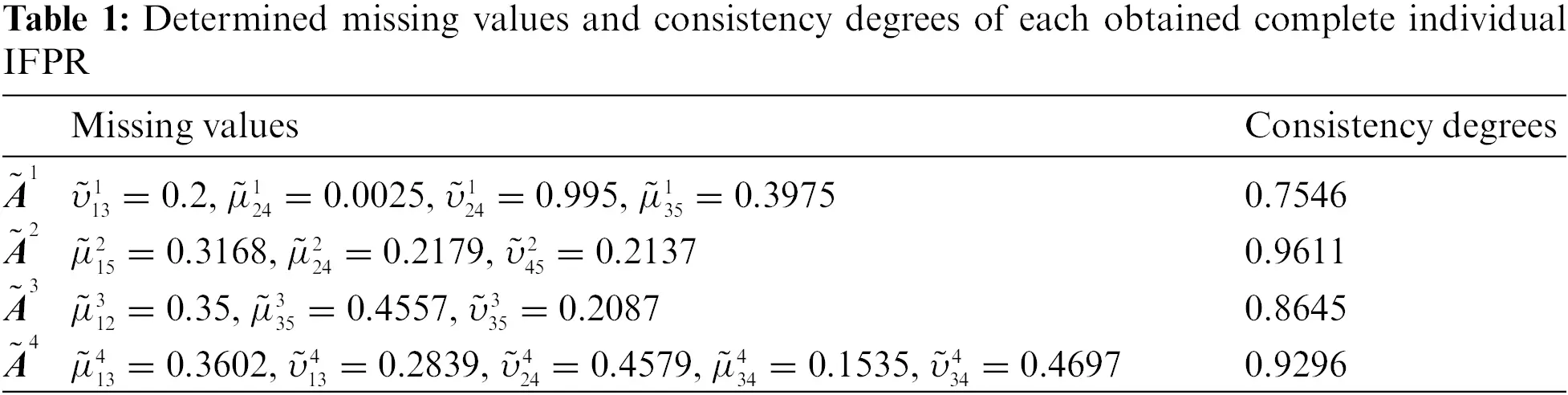Step 2: Use Eqs. (13)(17) to determine the experts’ weights as: λ1=0.2468, λ2=0.2537, λ3=0.2488, λ4=0.2507, and use Eq. (18) to generate the group IFPR A¯C as

A¯C=(0.5,0.50.3317,0.41090.398,0.35980.2296,0.44840.3912,0.40750.4109,0.33170.5,0.50.3649,0.31020.1739,0.57970.4495,0.3560.3598,0.3980.3102,0.36490.5,0.50.3147,0.460.3603,0.4030.4484,0.22960.5797,0.17390.46,0.31470.5,0.50.4398,0.3450.4075,0.39120.356,0.44950.403,0.36030.345,0.43980.5,0.5)

Step 3: Let CM0=0.888. Use Eq. (19) to calculate the consensus level of A¯h (h=1,2,3,4) as: GCM(A¯1)=0.877, GCM(A¯2)=0.9068, GCM(A¯3)=0.8632, and GCM(A¯4)=0.8823, which indicate that A¯2 achieves acceptable consensus, A¯h(h=1,3,4) are unacceptable consensus.

Step 4: Use Eqs. (21) and (22) to obtain the adjusted individual IFPRs Ah(h=1,2,3,4) and the group IFPR AC as: A2=A¯2, A4=A¯4,

A1=(0.5,0.50.2,0.30.764,0.20.4,0.30.6,0.250.3,0.20.5,0.50.2,0.40.1002,0.65690.5,0.20.2,0.7640.4,0.20.5,0.50.3,0.50.3975,0.60.3,0.40.6569,0.10020.5,0.30.5,0.50.451,0.33630.25,0.60.2,0.50.6,0.39750.3363,0.4510.5,0.5)A3=(0.5,0.50.35,0.40.348,0.4110.1,0.40.5,0.350.4,0.350.5,0.50.3,0.50.2,0.60.4495,0.3560.411,0.3480.5,0.30.5,0.50.7,0.20.456,0.20870.4,0.10.6,0.20.2,0.70.5,0.50.2,0.50.35,0.50.356,0.44950.2087,0.4560.5,0.20.5,0.5)AC=(0.5,0.50.3317,0.41090.4439,0.31720.2296,0.44840.3912,0.40750.4109,0.33170.5,0.50.3649,0.31020.1739,0.57970.4621,0.30130.3172,0.44390.3102,0.36490.5,0.50.3147,0.460.3603,0.4030.4484,0.22960.5797,0.17390.46,0.31470.5,0.50.4279,0.35440.4075,0.39120.3013,0.46210.403,0.36030.3544,0.42790.5,0.5)

Step 5: Use Eq. (27) to derive the group IFPWVs from AC as: w1C=0.1596,0.7862, w2C=0.1594,0.7298, w3C=0.1427,0.794, w4C=0.181,0.6107, w5C=0.149,0.7719.

Step 6: Calculate the similarity measures L(wiC) of wiC (i=1,2,3,4,5) by method  as L(w1C)=0.2028, L(w2C)=0.2432, L(w3C)=0.1938, L(w4C)=0.3222, L(w5C)=0.2114. Thus, the ranking order of alternatives is x4>x2>x5>x1>x3, and the best alternative is x4.

7.2 Sensitivity Analysis (SA)

Different values of tolerance parameters may bring diverse DM results. Therefore, it is necessary to conduct sensitivity analyses. Tables 2 and 3 represent the obtained missing values and the consistency degrees of the corresponding complete IFPRs under different values of tolerance parameters tikjh. From Tables 2 and 3, we find that all of missing values in A~1 obtained by Eq. (10) are basically identical under different values of tolerance parameters tikjh, and the missing values in A~i (i=2,3,4) obtained by Eq. (10) are significantly changed under different values of tolerance parameters tikjh. In addition, we also find that the consistency of the obtained corresponding complete IFPRs increases with the increase of the values of tolerance parameters tikjh.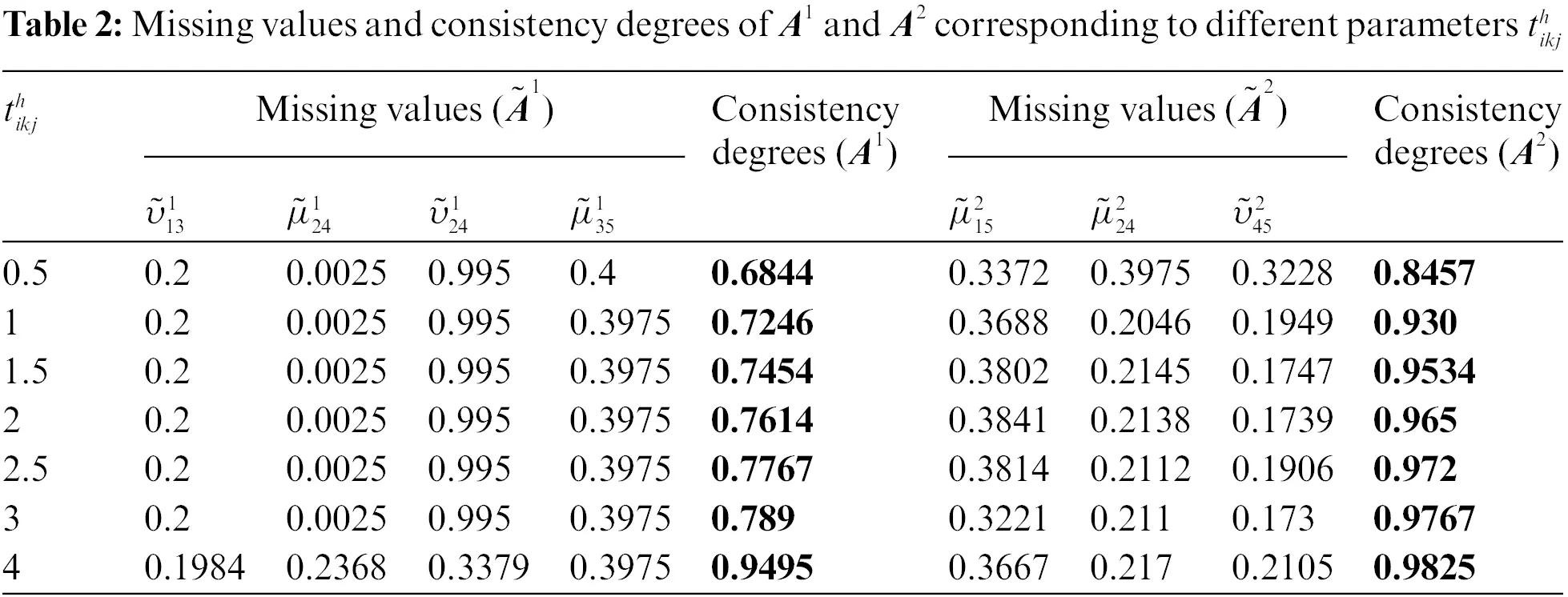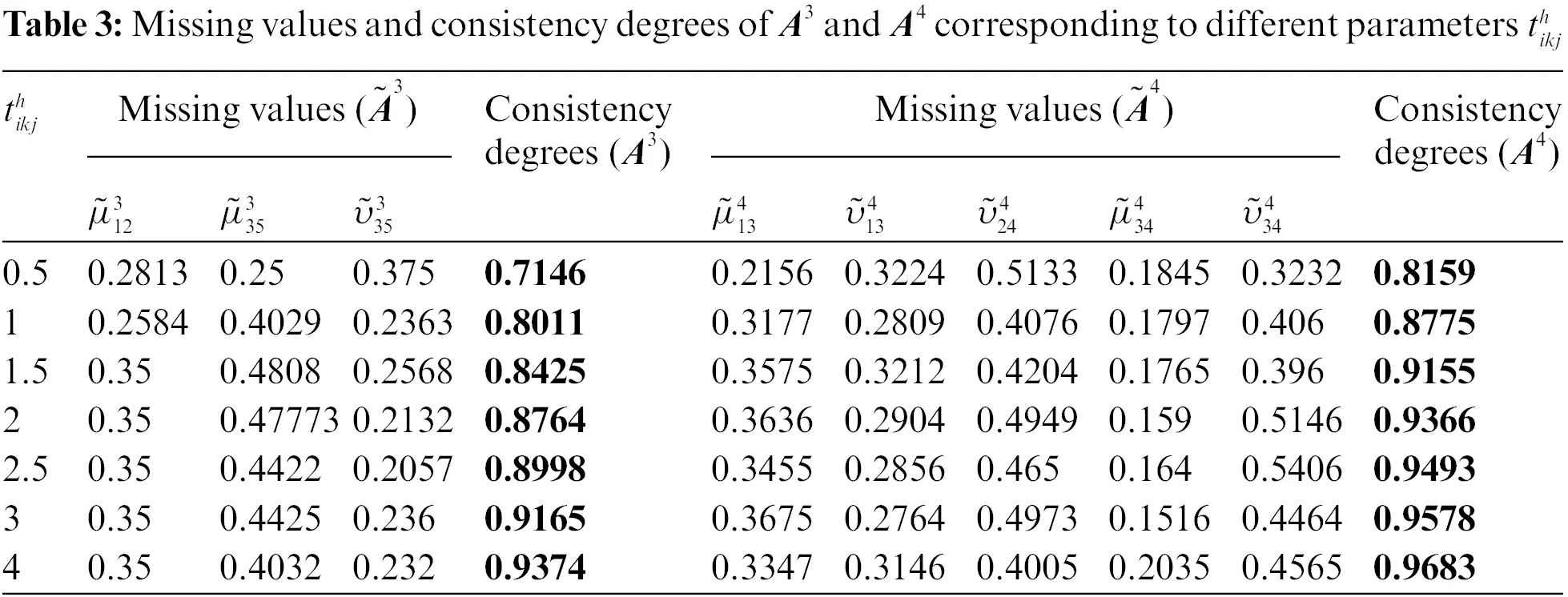Table 4 shows the related ranking values and orders of the alternatives respectively under different values of tolerance parameters tikjh (to ensure that Eq. (21)) has a feasible solution, assume that tikjh=2.5 in Eq. (21). In Table 4, we vary the values of tolerance parameters tikjh and again we observe that the best alternative is always x4. However, the ranking of alternatives changes with the change of tolerance parameters tikjh.

From Tables 2 and 3, we can conclude that experts can set different values of tolerance parameters tikjh according to their own preferences. If experts are conservative, they can set a smaller value tikjh. If experts are radical, they can set a larger a value tikjh. Thus, the GDM method proposed in this paper provides a more flexible and free DM space for experts to solve such GDM problems with incomplete intuitionistic fuzzy information.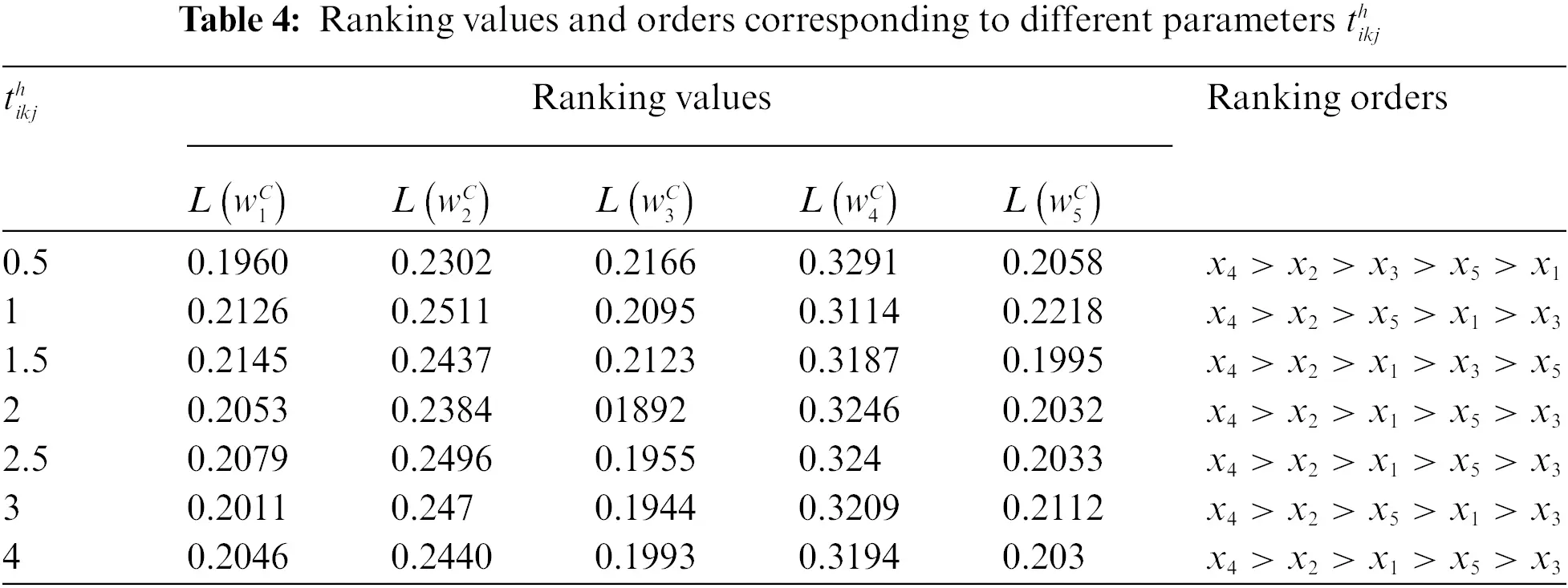7.3 Comparison Analyses with Existing GDM Methods with IFPRs

As for the existing GDM methods with IFPRs [24,2832,34], because Meng et al. conducted theoretical and numerical analyses in , we primarily compared the GDM method proposed in this study with the two methods mentioned in [29,34].

7.3.1 Comparative Analyses for GDM Methods with Complete IFPRs [29,34]

The GDM with a complete IFPR problem (Example 3) proposed in  aims to choose PhD students to study in overseas universities. Algorithm 2 is applied to handle the GDM problem presented in  (let tikjh=1.8(i,j=1,2,3,4;i<jki,j;hM), MCI0=0.9, and CM0=0.9). The ranking results of methods [29,34] and Algorithm 2 are listed in Table 5. From Table 5, we can see that the ranking orders of the alternatives obtained by Algorithm 2 and the methods [29,34] are identical, illustrating that Algorithm 2 is reasonable. Moreover, the information deviation between the original individual IFPRs and the adjusted individual IFPRs obtained by the method [29,34] and Algorithm 2 are calculated as shown in Table 6. From Table 6, it can be seen that the information deviation between the original individual IFPRs and the adjusted individual IFPRs obtained by Algorithm 2 is significantly lower than those obtained by method [29,34], indicating that Algorithm 2 has more advantages in preserving the original information than the method in [29,34].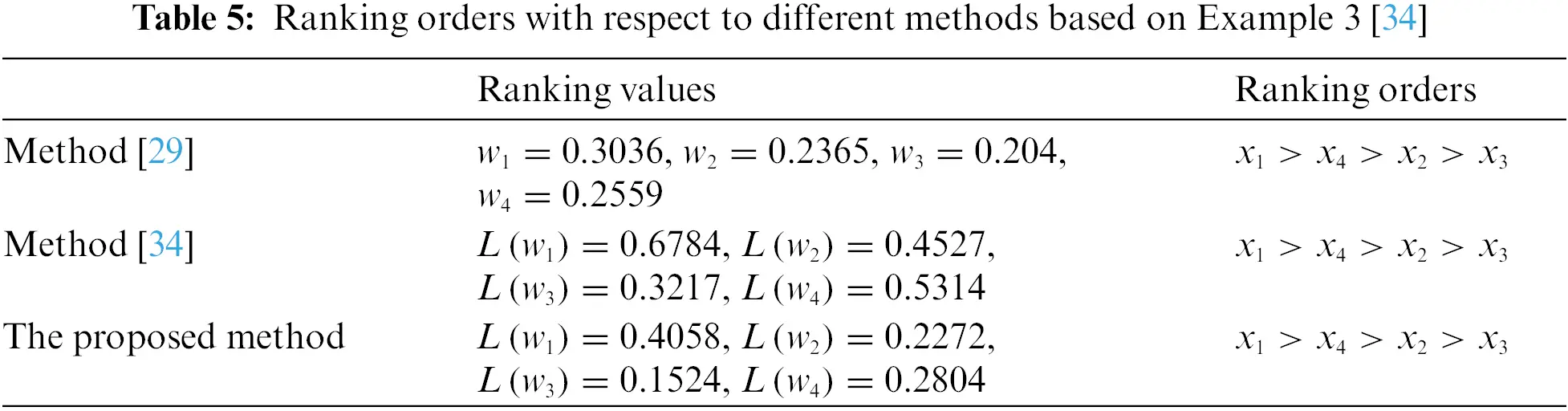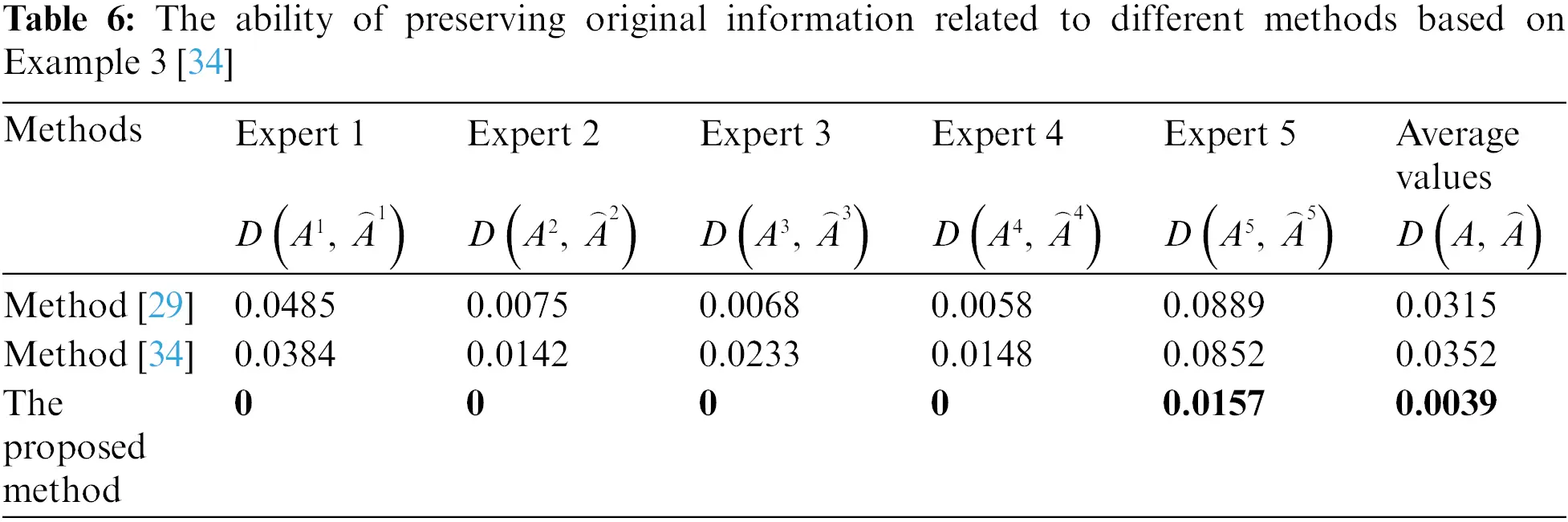7.3.2 Comparative Analyses for GDM Method with Incomplete IFPRs 

Algorithm 2 is used to handle the GDM problem (Example 4) with incomplete IFPRs, to illustrate the rationality and superiority of Algorithm 2 in solving the GDM problem with incomplete IFPRs, as shown in  (let tikjh=1.8, (i,j=1,2,3,4;i<jki,j;hM), MCI0=0.9, and CM0=0.9). Table 7 lists the missing values of each incomplete IFPR and the consistency degrees of the corresponding complete IFPRs obtained when Algorithm 2 is employed to solve Example 4 in . Tables 8 and 9 present the ranking orders and the ability to preserve the original information with respect to method  and Algorithm 2. From Table 8, it is evident that the ranking orders obtained by the method in  and Algorithm 2 are identical, which illustrates the rationality of Algorithm 2. However, Table 9 shows that Algorithm 2 has more advantages than method in  with regards to the ability to maintain the original information.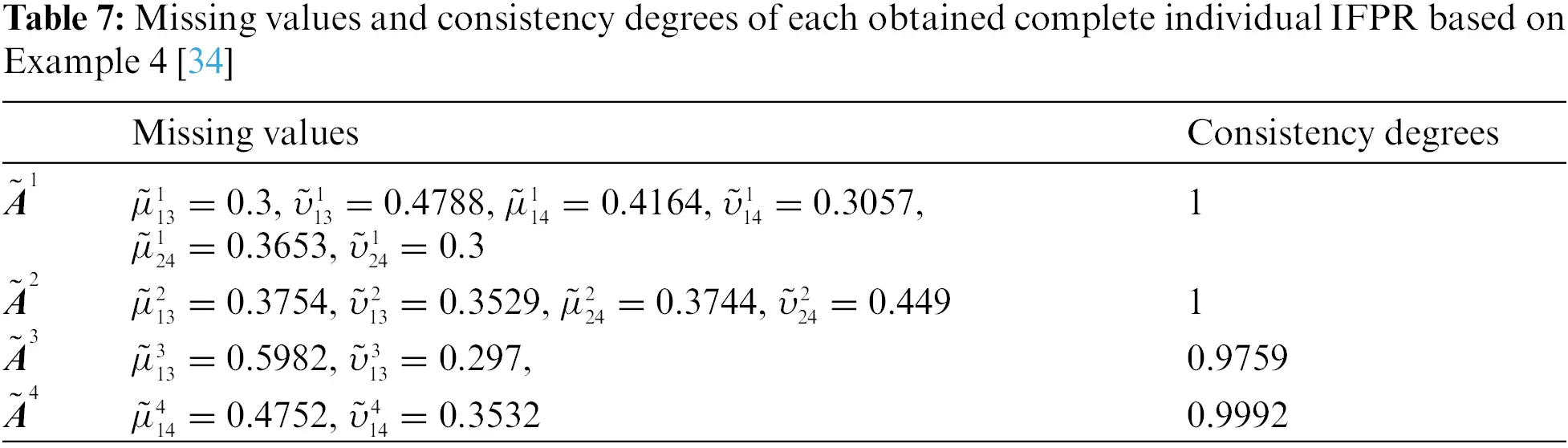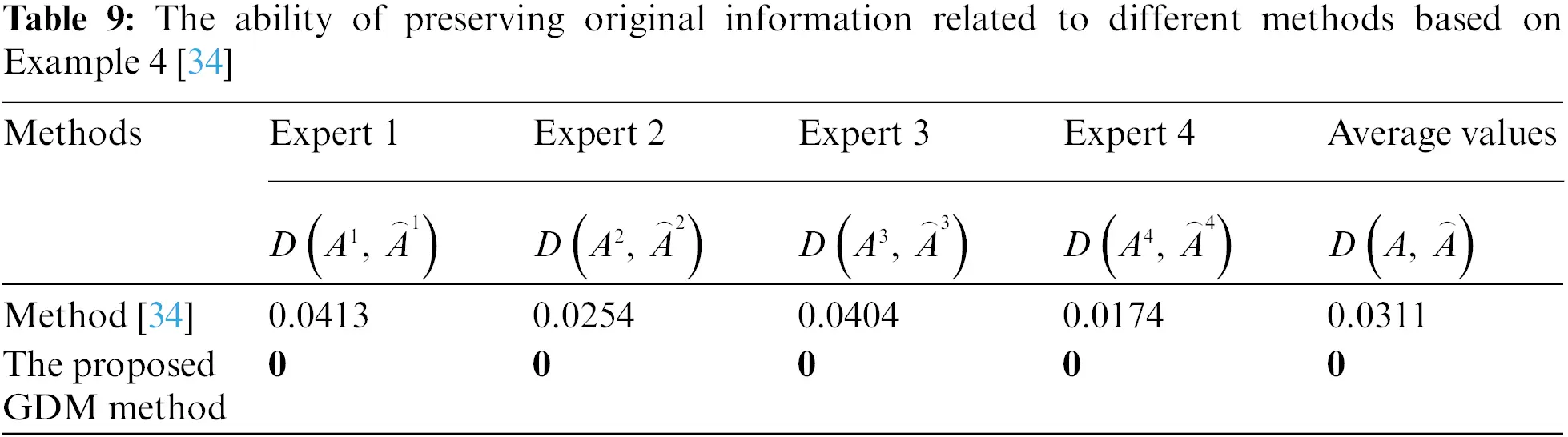From the above comparative analysis, the proposed method has the following advantages over the method in [29,34]:

(1) Compared with the consistency improvement framework proposed in [29,34], the framework proposed in this study is more reasonable. Considering the consistency improvement framework proposed in , as described in Section 4, since the multiplicative consistent definition for IFPRs presented in  is not a natural extension of Tanino’s multiplicative consistency definition of PRs, the obtained DM result based on the definition lacks a certain degree of persuasiveness. The consistency improvement framework introduced in  is based on the definition of complete consistency. However, owing to the difference in professional knowledge, it takes considerable time and energy to achieve the complete consistency of all experts. Meanwhile, the accomplishment of complete consistency is often at the cost of a considerable information loss, which can be found in the above examples.

(2) The CRP proposed in  adopts an optimization-based consensus to reach consensus among experts, because its adjustment is not targeted; that is, all individual IFPRs have been adjusted in this process, the final adjusted individual IFPRs may not be optimal in preserving the original information. For the CRP proposed in , although the individual IFPRs with the lowest level of consensus were selected and adjusted, its adjustment was targeted. However, the degree of retention of the original information is not considered in the adjustment process. In this study, we first select all individual IFPRs whose consensus is lower than the prescribed value and then adjust them through the optimization model to generate corresponding individual IFPRs satisfying the consensus requirements. Indeed, the method in this study combines the merits of the two methods, so it has more advantages in terms of consensus improvement over the method in [29,34].

(3) Compared with the method for determining the priority weights of alternatives proposed in [29,34], the method proposed in this study uses an optimization model to determine the priority weights of alternatives, whereas the methods in [29,34] used an aggregation operator to obtain the priority weights of alternatives. However, in certain situations, the use of these aggregation operators may lead to missing information. Thus, the method of determining the priority weights of alternatives proposed in this study is more convincing.

8  Conclusions

Therefore, considering the defects of existing GDM methods with incomplete IFPRs based on multiplicative consistency, this study develops a new method.

The contributions of this study are as follows: First, a new generalized multiplicative consistency for IFPRs is introduced, based on which two mathematical programming models are constructed to supplement the default values in incomplete IFPRs and improve the consistency level of unacceptably consistent IFPRs. Then, for GDM with incomplete IFPRs, a novel method for determining the weights of experts is introduced, and a targeted CRP to improve the consensus level of individual IFPRs is developed. Lastly, a new GDM with incomplete IFPRs is presented, the practicability of which is confirmed through an application example, and its superiority and rationality are explained through comparative and sensitivity analyses.

The benefits of this study are as follows. (1) The multiplicative consistency definition for IFPRs presented in this study, which compensates for the defects of the existing multiplicative consistency definitions for IFPRs, provides a somewhat theoretical guarantee for obtaining reasonable DM results. (2) The CRP presented in this study combines the merits of the existing CPR in GDM with IFPRs to enhance the efficiency of consensus and provides a certain theoretical guarantee for obtaining the DM result recognized by all experts. (3) The method using an optimization model to determine the priority weights of alternatives, avoids information loss, and provides a more convincing DM result.

The limitations of this study are as follows: since the decision data depend on the subjective information provided by the experts, the obtained DM result may have an element of inaccuracy and personal bias, and the consistency and consensus thresholds are provided in advance, which also lacks objectivity.

The implication of this study is that its findings can help community managers effectively reduce the confusion caused by chaotic parking in the community, thereby improving people’s quality of life. Moreover, the method proposed in this study can be applied to other real-world problems, such as supplier selection, risk evaluation, hotel location selection, etc.

Furthermore, the additive consistency of IFPRs is another tool used to evaluate the quality of pairwise comparisons of alternatives provided by the expert. Therefore, the application of our method to GDM problems based on additive consistent IFPRs is worth studying in the future. In addition, extending the method proposed in this study to other fuzzy environments, such as trapezoidal neutral data , m-polar set , nonlinear Pentagon intuitionistic fuzzy number , and intuitionistic multiplicative set [50,51], are also some interesting topics in the future.

Funding Statement: This research was supported by the National Natural Science Foundation of China (Nos. 71740021, 11861034, and 61966030), the Humanities Social Science Programming Project of Ministry of Education of China (No. 20YJA630059), the Natural Science Foundation of Jiangxi Province of China (No. 20192BAB207012), the Natural Science Foundation of Qinghai Province of China (No. 2019-ZJ-7086).

Conflicts of Interest: The authors declare that they have no conflicts of interest to report regarding the present study.

## References

1. Du, C., & Ye, J. (2021). Weighted parameterized correlation coefficients of indeterminacy fuzzy multisets and their multicriteria group decision making method with different decision risks. Computer Modeling in Engineering & Sciences, 129(1), 341-354. [Google Scholar] [CrossRef]
2. Ye, J. (2017). Bidirectional projection method for multiple attribute group decision making with neutrosophic numbers. Neural Computing and Applications, 28(5), 1021-1029. [Google Scholar] [CrossRef]
3. Luo, H. S., Li, L. J., Zhang, Y. K., Fang, S. Y., & Chen, X. Y. (2021). Link prediction in multiplex networks using a novel multiple-attribute decision-making approach. Knowledge-Based Systems, 219(11), 106904. [Google Scholar] [CrossRef]
4. Wan, S. P., Wang, F., & Dong, J. Y. (2016). A novel group decision making method with intuitionistic fuzzy preference relations for RFID technology selection. Applied Soft Computing, 38(2), 405-422. [Google Scholar] [CrossRef]
5. Zhang, Z. M., & Chen, X. M. (2021). Group decision making based on multiplicative consistency and consensus preference analysis for incomplete -rung orthopair fuzzy preference relations. Information Sciences, 574(7), 653-673. [Google Scholar] [CrossRef]
6. Saaty, T. L. (1986). Axiomatic foundation of the analytic hierarchy process. Management Science, 32(7), 841-855. [Google Scholar] [CrossRef]
7. Orlovsky, S. A. (1978). Decision-making with a fuzzy preference relation. Fuzzy Sets and Systems, 1(3), 155-167. [Google Scholar] [CrossRef]
8. Chiclana, F., Herrera, F., & Herrera-Viedma, E. (2001). Integrating multiplicative preference relations in a multipurpose decision-making model based on fuzzy preference relations. Fuzzy Sets and Systems, 122(2), 277-291. [Google Scholar] [CrossRef]
9. Xu, Z. S., & Chen, J. (2008). Some models for deriving the priority weights from interval fuzzy preference relations. European Journal of Operational Research, 184(1), 266-280. [Google Scholar] [CrossRef]
10. Wan, S. P., Wang, F., & Dong, J. Y. (2018). A group decision making method with interval valued fuzzy preference relations based on the geometric consistency. Information Fusion, 40(1), 87-100. [Google Scholar] [CrossRef]
11. Xu, Z. S. (2007). Intuitionistic preference relations and their application in group decision making. Information Sciences, 177(11), 2363-2379. [Google Scholar] [CrossRef]
12. Garg, H. (2018). Generalized interaction aggregation operators in intuitionistic fuzzy multiplicative preference environment and their application to multicriteria decision-making. Applied Intelligence, 48(8), 2120-2136. [Google Scholar] [CrossRef]
13. Rani, D., & Garg, H. (2021). Complex intuitionistic fuzzy preference relations and their applications in individual and group decision-making problems. International Journal of Intelligent Systems, 36(4), 1800-1830. [Google Scholar] [CrossRef]
14. Wan, S. P., Zhong, L. G., & Dong, J. Y. (2020). A new method for group decision making with hesitant fuzzy preference relations based on multiplicative consistency. IEEE Transactions on Fuzzy Systems, 28(7), 1449-1463. [Google Scholar] [CrossRef]
15. Jin, F. F., Garg, H., Pei, L. D., Liu, J. P., & Chen, H. Y. (2020). Multiplicative consistency adjustment model and data envelopment analysis-driven decision-making process with probabilistic hesitant fuzzy preference relations. International Journal of Fuzzy Systems, 22(7), 2319-2332. [Google Scholar] [CrossRef]
16. Garg, H. (2022). SVNMPR: A new single-valued neutrosophic multiplicative preference relation and their application to decision-making process. International Journal of Intelligent Systems, 37(3), 2089-2130. [Google Scholar] [CrossRef]
17. Atanassov, K. (1986). Intuitionistic fuzzy sets. Fuzzy Sets and Systems, 20(1), 87-96. [Google Scholar] [CrossRef]
18. Liao, H. C., & Xu, Z. S. (2014). Multi-criteria decision making with intuitionistic fuzzy PROMETHEE. Journal of Intelligent & Fuzzy Systems, 27(4), 1703-1717. [Google Scholar] [CrossRef]
19. Liao, H. C., Mi, X. M., Xu, Z. S., Xu, J. P., & Herrera, F. (2018). Intuitionistic fuzzy analytic network process. IEEE Transactions on Fuzzy Systems, 26(5), 2578-2590. [Google Scholar] [CrossRef]
20. Wan, S. P., & Dong, J. Y. (2021). A novel extension of best-worst method with intuitionistic fuzzy reference comparisons. IEEE Transactions on Fuzzy Systems, 30(6), 1698-1711. [Google Scholar] [CrossRef]
21. Efe, B. (2019). Analysis of operational safety risks in shipbuilding using failure mode and effect analysis approach. Ocean Engineering, 187(4), 106214. [Google Scholar] [CrossRef]
22. Stanujkić, D., & Karabašević, D. (2018). An extension of the WASPAS method for decision-making problems with intuitionistic fuzzy numbers: A case of website evaluation. Operational Research in Engineering Sciences: Theory and Applications, 1(1), 29-39. [Google Scholar] [CrossRef]
23. Kushwaha, D. K., Panchal, D., & Sachdeva, A. (2020). Risk analysis of cutting system under intuitionistic fuzzy environment. Reports in Mechanical Engineering, 1(1), 162-173. [Google Scholar] [CrossRef]
24. Xu, Z. S., & Yager, R. R. (2009). Intuitionistic and interval-valued intutionistic fuzzy preference relations and their measures of similarity for the evaluation of agreement within a group. Fuzzy Optimization and Decision Making, 8(2), 123-139. [Google Scholar] [CrossRef]
25. Xu, Z. S., Cai, X. Q., & Szmidt, E. (2011). Algorithms for estimating missing elements of incomplete intuitionistic preference relations. International Journal of Intelligent Systems, 9(26), 787-813. [Google Scholar] [CrossRef]
26. Xu, Z. S., & Liao, H. C. (2014). Intuitionistic fuzzy analytic hierarchy process. IEEE Transactions on Fuzzy Systems, 22(4), 749-761. [Google Scholar] [CrossRef]
27. Liao, H. C., & Xu, Z. S. (2014). Priorities of intuitionistic fuzzy preference relation based on multiplicative consistency. IEEE Transactions on Fuzzy Systems, 22(6), 1669-1681. [Google Scholar] [CrossRef]
28. Liao, H. C., Xu, Z. S., Zeng, X. J., & Merigó, J. M. (2015). Framework of group decision making with intuitionistic fuzzy preference information. IEEE Transactions on Fuzzy Systems, 23(4), 1211-1227. [Google Scholar] [CrossRef]
29. Xu, G. L., Wan, S. P., Wang, F., Dong, J. Y., & Ze, Y. F. (2016). Mathematical programming methods for consistency and consensus in group decision making with intuitionistic fuzzy preference relations. Knowledge-Based Systems, 98, 30-43. [Google Scholar] [CrossRef]
30. Jin, F. F., Ni, Z. W., Chen, H. Y., & Li, Y. P. (2016). Approaches to group decision making with in intuitionistic fuzzy preference relations based on multiplicative consistency. Knowledge-Based Systems, 97(1), 48-59. [Google Scholar] [CrossRef]
31. Gong, Z. W., Li, L. S., Zhou, F. X., & Yao, T. X. (2009). Goal programming approaches to obtain the priority vectors from the intuitionistic fuzzy preference relations. Computers & Industrial Engineering, 57(4), 1187-1193. [Google Scholar] [CrossRef]
32. Wu, J., & Chiclana, F. (2014). Multiplicative consistency of intuitionistic reciprocal preference relations and its application to missing values estimation and consensus building. Knowledge-Based Systems, 71(1), 187-200. [Google Scholar] [CrossRef]
33. Wu, J., Chiclana, F., & Liao, H. C. (2018). Isomorphic multiplicative transitivity for intuitionistic and interval-valued fuzzy preference relations and its application in deriving their priority vectors. IEEE Transactions on Fuzzy Systems, 26(1), 193-202. [Google Scholar] [CrossRef]
34. Meng, F. Y., Tang, J., & Xu, Z. S. (2017). A 0-1 mixed programming model based method for group decision making with intuitionistic fuzzy preference relations. Computers & Industrial Engineering, 112, 289-304. [Google Scholar] [CrossRef]
35. Krejčí, J. (2017). On multiplicative consistency of interval and fuzzy reciprocal preference relations. Computers & Industrial Engineering, 111(3), 67-78. [Google Scholar] [CrossRef]
36. Meng, F. Y., Chen, S. M., & Yuan, R. P. (2020). Group decision making with heterogeneous intuitionistic fuzzy preference relations. Information Sciences, 523(1), 197-219. [Google Scholar] [CrossRef]
37. Wang, Z. J. (2020). A representable uninorm based intuitionistic fuzzy analytic hierarchy process. IEEE Transactions on Fuzzy Systems, 28(10), 2555-2569. [Google Scholar] [CrossRef]
38. Xu, Z. S., & Yager, R. R. (2006). Some geometric aggregation based on intuitionistic fuzzy sets. International Journal of General Systems, 35(4), 417-433. [Google Scholar] [CrossRef]
39. Szmidt, E., & Kacprzyk, J. (2000). Distances between intuitionistic fuzzy sets. Fuzzy Sets and Systems, 114(3), 505-518. [Google Scholar] [CrossRef]
40. Tanino, T. (1984). Fuzzy preference orderings in group decision making. Fuzzy Sets and Systems, 12(2), 117-131. [Google Scholar] [CrossRef]
41. Palomares, I., Estrella, F. J., Martínez, L., & Herrera, F. (2014). Consensus under a fuzzy context: Taxonomy, analysis framework AFRYCA and experimental case of study. Information Fusion, 20(2), 252-271. [Google Scholar] [CrossRef]
42. Herrera, F., Herrera-Viedma, E., & Verdegay, J. (1996). A model of consensus in group decision making under linguistic assessments. Fuzzy Sets and Systems, 78(1), 73-87. [Google Scholar] [CrossRef]
43. Herrera, F., Herrera-Viedma, E., & Verdegay, J. (1997). Linguistic measures based on fuzzy coincidence for reaching consensus in group decision making. International Journal of Approximate Reasoning, 16(3–4), 309-334. [Google Scholar] [CrossRef]
44. Xu, Z. S., & Xia, M. M. (2014). Iterative algorithms for improving consistency of intuitionistic preference relations. Journal of the Operational Research Society, 65(5), 708-722. [Google Scholar] [CrossRef]
45. Wang, Z. J. (2013). Derivation of intuitionistic fuzzy weights based on intuitionistic fuzzy preference relations. Applied Mathematical Modelling, 37(9), 6377-6388. [Google Scholar] [CrossRef]
46. Zhang, X. M., & Xu, Z. S. (2012). A new method for ranking intuitionistic fuzzy values and its application in multi-attribute decision making. Fuzzy Optimization and Decision Making, 11(2), 135-146. [Google Scholar] [CrossRef]
47. Haque, T. S., Chakraborty, A., Mondal, S. P., & Alam, S. (2021). New exponential operational law for measuring pollution attributes in mega-cities based on MCGDM problem with trapezoidal neutrosophic data. Journal of Ambient Intelligence and Humanized Computing, 77(4), 438. [Google Scholar] [CrossRef]
48. Hashmi, M. R., Riaz, M., & Smarandache, F. (2020). m-polar neutrosophic topology with applications to multi-criteria decision-making in medical diagnosis and clustering analysis. International Journal of Fuzzy Systems, 22(1), 273-292. [Google Scholar] [CrossRef]
49. Chakraborty, A., Pal, S., Mondal, S. P., & Alam, S. (2021). Nonlinear pentagonal intuitionistic fuzzy number and its application in EPQ model under learning and forgetting. Complex & Intelligent Systems, 8(2), 1307-1322. [Google Scholar] [CrossRef]
50. Garg, H. (2020). Exponential operational laws and new aggregation operators for intuitionistic multiplicative set in multiple-attribute group decision making process. Information Sciences, 538(1), 245-272. [Google Scholar] [CrossRef]
51. Garg, H. (2021). Multi-attribute group decision-making process based on possibility degree and operators for intuitionistic multiplicative set. Complex and Intelligent Systems, 7(2), 1099-1121. [Google Scholar] [CrossRef]This work is licensed under a Creative Commons Attribution 4.0 International License, which permits unrestricted use, distribution, and reproduction in any medium, provided the original work is properly cited.